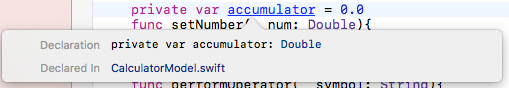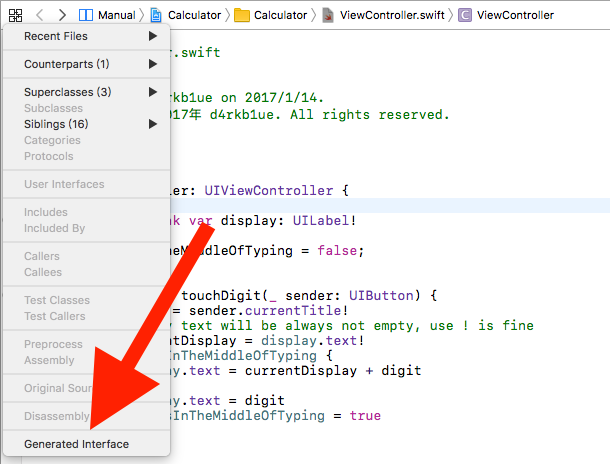• Computed Property
• Apply the MVC
• Struct
• Closure
• Enumerations

# Computed Property

``````var displayValue: Double {
get{
return Double(display.text!)!
}
set{
display.text = String(newValue)
}
``````

it’s a var. but contains some functions. `newValue` is a pre-defined keyword.

When we call

``````displayValue = some_value
``````

aka, the `displayValue` is being SET the function `set` well be executed. Similarly, when we call

``````new_some_value = displayValue
``````

aka, the `displayValue` is being GET, the function `get` will be executed; or like `func(displayValue)`.

if we don’t specify a `set`, this property will be READ-ONLY; if no `get`, it will be WRITE-ONLY;

So the computed property react with the sign `=`. `==` or `<`, `>` will not call the function.

# Apply the MVC

• Model: the datas, algorithms, calculations; don’t know the view;
• View: interact with users, show things to them; don’t know the model;
• Controller: listen to the models, tell the models; interact with view;

## Model

new file, iOS, swift, name it “CalculatorModel”.

create a new object:

``````private var model = CalculatorModel()
``````

since it is `= CalculatorModel()` , we do not need to specify `model: Calculator`; the same as, `var kkk: Double = 0.0;` simply, it can be `var kkk = 0.0`; the Xcode can refer it as a `Double`:if `var i = 0` it will be refer to an `Int`

### authority

Private: make func/var private firstly. If need, change them to public, not the opposite. Show class’s interface:like this:

``````internal class ViewController : UIViewController {

@IBOutlet weak internal var display: UILabel!

internal var userIsInTheMiddleOfTyping: Bool

@IBAction internal func touchDigit(_ sender: UIButton) -> <<error type>>

internal var displayValue: Double { get set }

@IBAction internal func performOperation(_ sender: UIButton) -> <<error type>>
}
``````

Diff: `internel` & `public`

• `internal` is public in the module; since we have only one app, the internal basically is the `public`
• `public` is public in other modules; like `UIkit`, it has many public methods, but also a lot of internal methods which can be called between themselves in the `UIkit`

reformat(indent): Ctrl + I

about `var`:

• if it start with small case, it will be a local variable: `var localVar`
• otherwise, a public variable: `var PublicVar`
• also, always use Camel Case(驼峰)

# Enumerations

## Example

Define:

``````enum CompassPoint {
case north
case south
case east
case west
}
// in a line
enum Planet {
case mercury, venus, earth, mars, jupiter, saturn, uranus, neptune
}
``````

Usage:

``````var directionToHead = CompassPoint.west

``````

Once the variable is defined by the enum type, next time, things will be simpler. (also when the Xcode can guess this type)

use with `switch`

``````directionToHead = .south
case .north:
print("Lots of planets have a north")
case .south:
print("Watch out for penguins")
case .east:
print("Where the sun rises")
case .west:
print("Where the skies are blue")
// since we cover all the case define in the enum, we do not need
// default: ...
}
``````

since we cover all the case define in the enum, we do not need a `default: …`; but this do not mean we must be exhaustive, omit some cases and provide a `default` is acceptable.

## Associated Values

it is sometimes useful to be able to store associated values of other types alongside these case values. This enables you to store additional custom information along with the case value, and permits this information to vary each time you use that case in your code.

``````enum Barcode {
case upc(Int, Int, Int, Int)
case qrCode(String)
}
``````

define the enum type, must have an associated value.

usage:

``````var productBarcode = Barcode.upc(8, 85909, 51226, 3)
// once the type is settled, the enum name can be ignored
productBarcode = .qrCode("ABCDEFGHIJKLMNOP")
``````

use with `switch`:

``````switch productBarcode {
case .upc(let numberSystem, let manufacturer, let product, let check):
print("UPC: \(numberSystem), \(manufacturer), \(product), \(check).")
case .qrCode(let productCode):
print("QR code: \(productCode).")
}
``````

all the associated values can be `let` to get the value. it can be write like this: `case let .upc(numberSystem, manufacturer, product, check):` (= `case .upc(let numberSystem, let manufacturer, let product, let check)`)

Actually, `optional`, aka, `TYPE ?`is an enum.

``````enum Optional<T> {
case None
case Some<T>
}
``````

# Closure

``````private func multiply(op1: Double, op2: Double) -> Double {
return op1 * op2
}
``````

Function is a type of value. Same as Double or String. Most important, it can has its own attributes when claim:

``````private func passAFunction((Double, Double) —> Double) {
...
}
// call it
passAFunction(multiply)
``````

function `multiply` is not decent, it can be turned like this:

``````// need a "in" between declaration and statement
passAFuction(
{ (op1: Double, op2: Double) -> Double in
return op1 * op2
}
)
``````

because Swift know that `passAFunction ` need a `(Double, Double) —> Double)` , we can proceed,

``````passAFunction(
{ (op1, op2) in
return op1 * op2
}
)
``````

Closure has default value name, `\$0` for the 1st parameter, `\$1` for the 2nd, `\$2` for the 3rd and go on. So, proceed,

``````passAFunction(
{
return \$0 * \$1
}
)
``````

Moreover,

``````passAFunction({ \$0 * \$1 } )
``````

another example:

``````[...
"±" : Operation.UnaryOp({ -\$0 }),
...]
``````

# Struct

Struct is very similar to Class. Diff Struct & Class

• Can not inherit
• Pass value by value, not reference(Struct always get copied)
• Struct’s default constructer function contains all the parameters, while Class has none

In fact, a passed Struct not always get copied unless the new reference get touched.

# Questions!

## function as parameter

if I put

``````private func multiply(op1: Double, op2: Double) -> Double {
return op1 * op2
}
``````

inside the Class like:

``````class CalculatorModel {
private func multiply(op1: Double, op2: Double) -> Double {
return op1 * op2
}

var operations: Dictionary<String, Operation> = [
"×" : Operation.BinaryOp(multiply)
]
``````

and it’s just wrong!

``````Cannot convert value of type '(CalculatorModel) -> (Double, Double) -> Double' to expected argument type '(Double, Double) -> Double'
``````

but when I put the multiply function out of the Class. It turns OK.